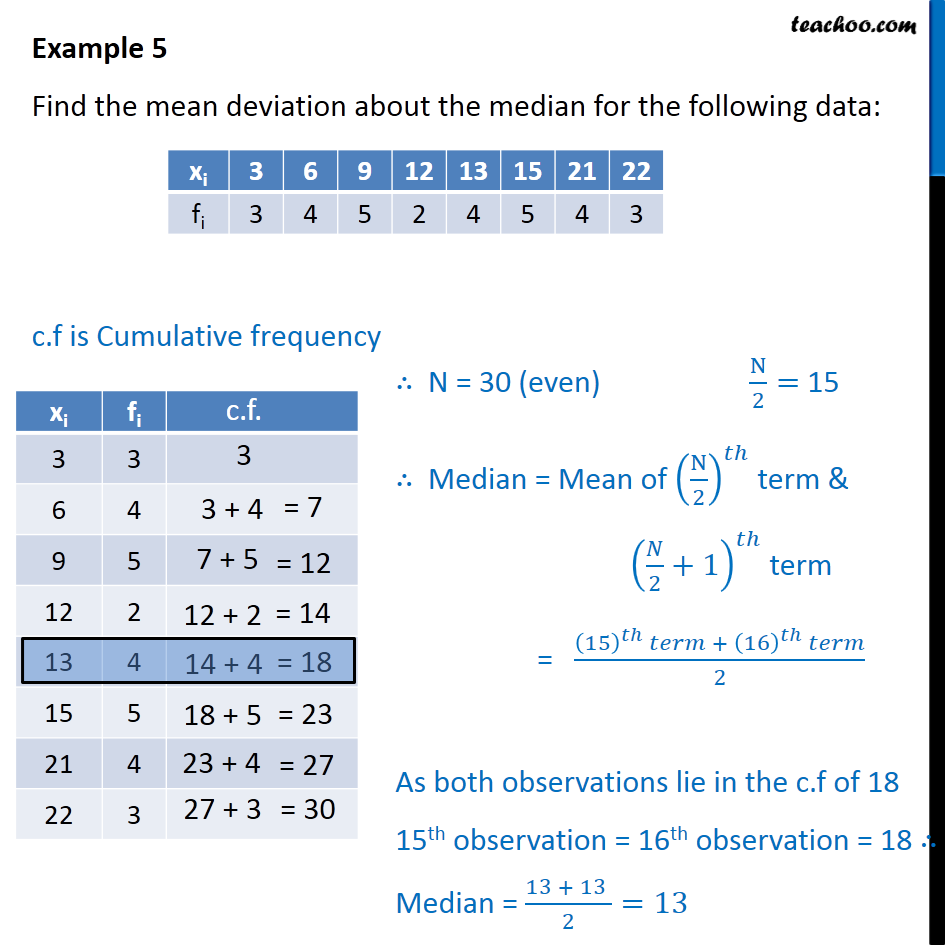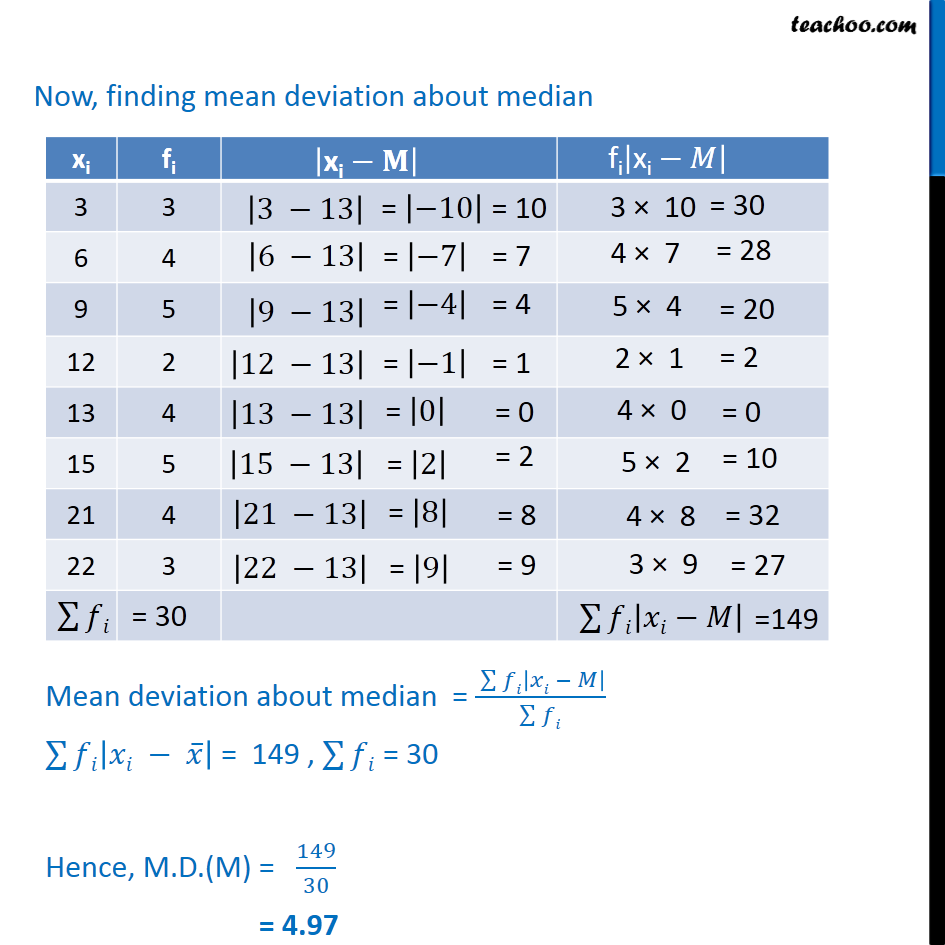Examples

Chapter 13 Class 11 Statistics
Serial order wiseLearn in your speed, with individual attention - Teachoo Maths 1-on-1 Class

### Transcript

Example 5 - Chapter 15 Class 11 Statistics - NCERT Find the mean deviation about the median for the following data: xi 3 6 9 12 13 15 21 22 fi 3 4 5 2 4 5 4 3 c.f is Cumulative frequency N = 29 (odd) ∴ Median = ((𝑁 + 1)/2)^𝑡ℎterm = ((29 + 1)/2)^𝑡ℎ term = (30/2)^𝑡ℎ term = 15th term Now, finding mean deviation about median As, 15th term lie in c.f of 21. Median = 30 ∑128▒𝑓𝑖 = 29 ∑128▒𝑓𝑖 |𝑥𝑖 −M| = 148 ∴ Mean Deviation = (Σ 𝑓_𝑖 |𝑥_(𝑖 )− M|)/𝑓_𝑖 = 148/29 = 5.1